## Use the quadratic formula to find the solution to the quadratic equation given below. X^2-x+1/4=0

Question

Use the quadratic formula to find the solution to the quadratic equation given
below.
X^2-x+1/4=0

in progress 0
3 months 2021-07-24T17:51:21+00:00 1 Answers 5 views 0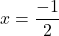General Formulas and Concepts:

Pre-Algebra

Order of Operations: BPEMDAS

1. Brackets
2. Parenthesis
3. Exponents
4. Multiplication
5. Division
7. Subtraction
• Left to Right

Algebra I

• Standard Form: ax² + bx + c = 0
• Quadratic Formula: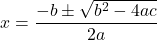Step-by-step explanation:

Step 1: Define

Identify

x² + x + 1/4 = 0

↓ Compare to Standard Form

a = 1, b = 1, c = 1/4

Step 2: Solve for x

1. Substitute in variables [Quadratic Formula]: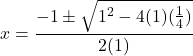2. [√Radical] Evaluate exponents: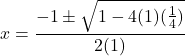3. [√Radical] Multiply: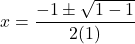4. [√Radical] Subtract: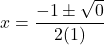5. [√Radical] Evaluate: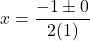6. Simplify: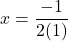7. Multiply: